##Cosymmedian triangle

Triangle DEF is the cosymmedian triangle of ABC. Its vertices D, E, F are the intersections of the symmedians of ABC with the circumcircle c of ABC. K is the symmedian or Lemoine point of triangle ABC. Triangles ABC and DEF share the same Lemoine point K, same Brocard circle with diameter OK, circumcircle c, Lemoine axis A*B*C*, Brocard axis (line [OK]), isodynamic points and Apollonian circles.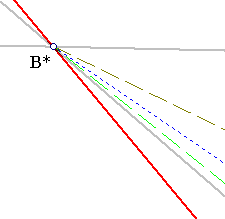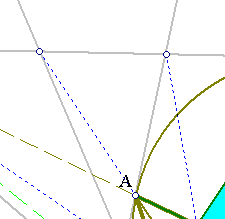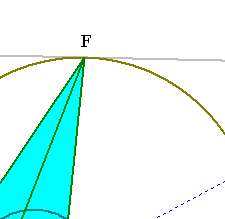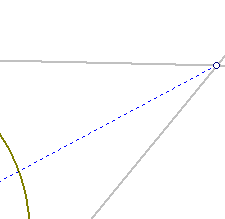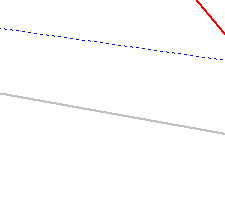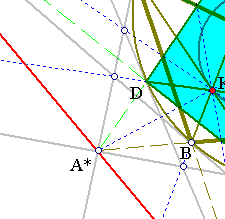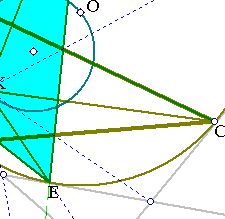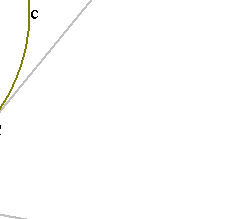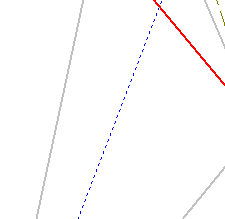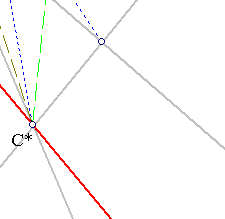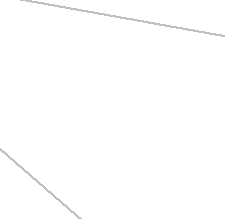All these are consequences of the fact that the cosymmedian triangle is an orbit of the (Moebius) Recycler of ABC. This is studied in Recycler.html .

 Produced with EucliDraw© http://users.math.uoc.gr/~pamfilos/